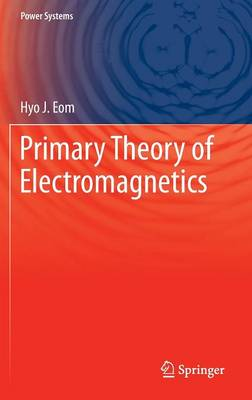•Primary Theory of Electromagnetics - Power Systems (Hardback)

(author)
£69.99
Hardback 205 Pages / Published: 17/09/2013
• We can order this

Usually dispatched within 3 weeks

This is a textbook on electromagnetics for undergraduate students in electrical engineering, information, and communications. The book contents are very compact and brief compared to other commonly known electromagnetic books for undergraduate students and emphasizes mathematical aspects of basic electromagnetic theory. The book presents basic electromagnetic theory starting from static fields to time-varying fields. Topics are divided into static electric fields, static magnetic fields, time-varying fields, and electromagnetic waves. The goal of this textbook is to lead students away from memorization, but towards a deeper understanding of formulas that are used in electromagnetic theory. Many formulas commonly used for electromagnetic analysis are mathematically derived from a few empirical laws. Physical interpretations of formulas are de-emphasized. Each important formula is framed to indicate its significance. Primary Theory of Electromagnetics shows a clear and rigorous account of formulas in a consistent manner, thus letting students understand how electromagnetic formulas are related to each other.

Publisher: Springer
ISBN: 9789400771420
Number of pages: 205
Weight: 4498 g
Dimensions: 235 x 155 x 14 mm
Edition: 2013 ed.